How to Learn in 24 Hours?The Rapid Learning Movie

 Need Help? M-F: 9am-5pm(PST): Toll-Free: (877) RAPID-10 US Direct: (714) 692-2900 Int'l: 001-714-692-2900 24/7 Online Technical Support: The Rapid Support Center Secure Online Order:Got Questions? Frequently Asked Questions
 Need Proof? Testimonials by Our Users
 Trustlink is a Better Business Bureau Program. Rapid Learning Center is a fivr-star business. External TrustLink Reviews

 Rapid Learning Courses: MCAT in 24 Hours (2015-16) USMLE in 24 Hours (Boards) Chemistry in 24 Hours Biology in 24 Hours Physics in 24 Hours Mathematics in 24 Hours Psychology in 24 Hours SAT in 24 Hours ACT in 24 Hours AP in 24 Hours CLEP in 24 Hours DAT in 24 Hours (Dental) OAT in 24 Hours (Optometry) PCAT in 24 Hours (Pharmacy) Nursing Entrance Exams Certification in 24 Hours eBook - Survival Kits Audiobooks (MP3)

 Tell-A-Friend: Have friends taking science and math courses too? Tell them about our rapid learning system.Home »  Mathematics »  High School Algebra 2

 Topic Review on "Title": Quadratic Equation: A quadratic equation is a specific type of equation written on the standard form: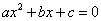where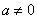. The Zero Product Rule: If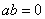, then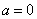or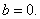Square Root Method: If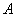and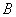are algebraic expressions such that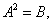then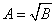or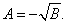Completing the Square Method: Completing the square consists of adding to both sides of an equation a positive number that can help factor a quadratic equation. Quadratic Formula: The solution of a quadratic equation of the formdepends on the sign of the discriminant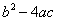. Quadratic Inequality: A quadratic inequality is a quadratic equation where the equality sign is replaced by an inequality. Quadratic Function: A quadratic function is a function where for each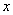the real number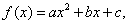a, b, and c are real numbers with a not equal to 0. Parabola: A parabola is the graph of a quadratic function. Vertex: A vertex is the point where the parabola intersects the axis of symmetry.

Rapid Study Kit for "Title":
 Flash Movie Flash Game Flash Card Core Concept Tutorial Problem Solving Drill Review Cheat Sheet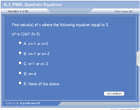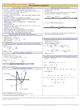"Title" Tutorial Summary : This tutorial describes the properties of quadratic functions and what they are. Quadratic equations are solved using the square root method. Completing the square of a quadratic equation is shown in some of the examples. The use of the quadratic formula is discussed in some of the examples. The difference between quadratic equations and quadratic inequalities is shown in some of the examples. Quadratic inequality solving techniques are mentioned in some of the examples. Finally, the use of the quadratic equation graphs is shown in some examples. The vertex and the axis of symmetry are defined with the use graphical examples

 Tutorial Features: Specific Tutorial Features: • Step by step examples are shown to introduce quadratic equations, square root method, and graphs of quadratic functions. • The quadratic equation and the quadratic formula are shown with the use of examples. Concept questions are asked to test the important ideals of quadratic equations. Series Features: • Concept map showing inter-connections of new concepts in this tutorial and those previously introduced. • Definition slides introduce terms as they are needed. • Visual representation of concepts • Animated examples—worked out step by step • A concise summary is given at the conclusion of the tutorial.

 "Title" Topic List: Quadratic Equations Definition of a quadratic equation Solving a quadratic equation Square Root Method Completing the Square Method Quadratic Formula Solving Quadratic FormulasQuadratic Inequalities Definition of quadratic inequalities Solving quadratic inequalities with roots or no rootsQuadratic Functions Definition of quadratic functions

See all 24 lessons in High School Algebra 2, including concept tutorials, problem drills and cheat sheets:
Teach Yourself High School Algebra 2 Visually in 24 Hours